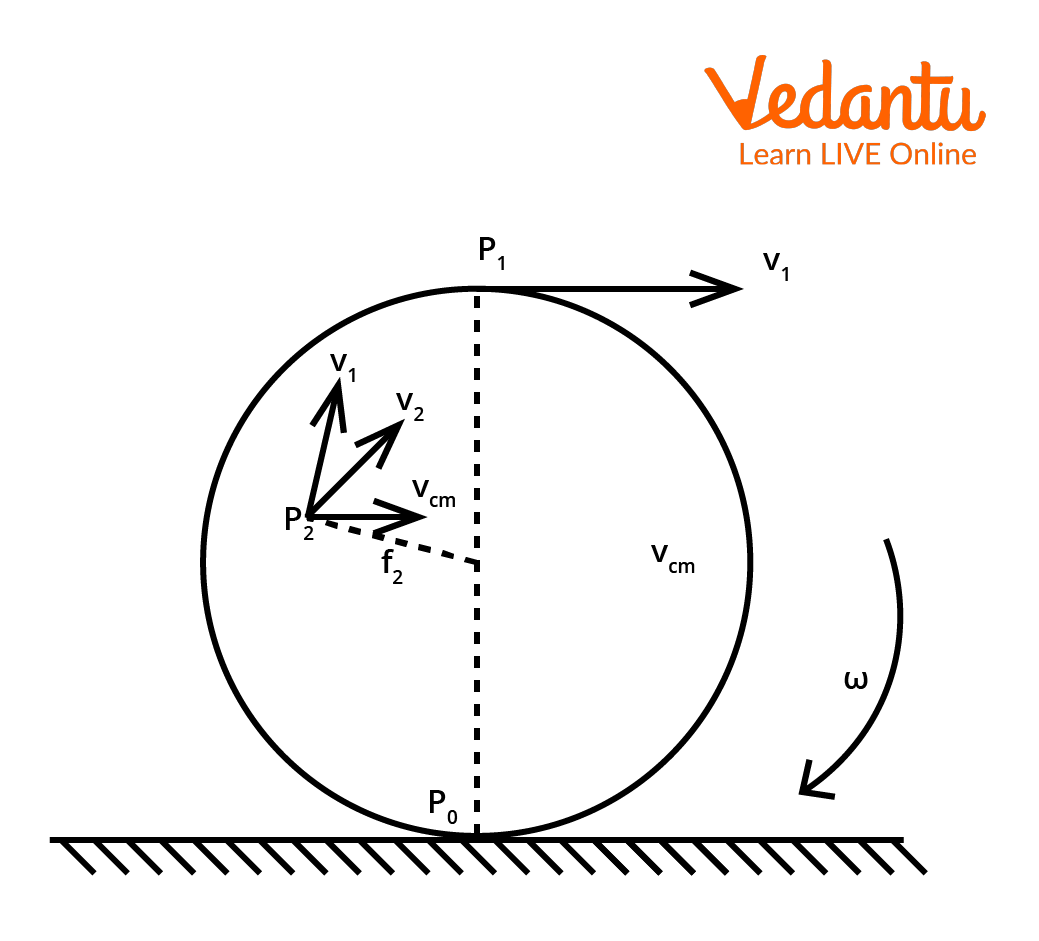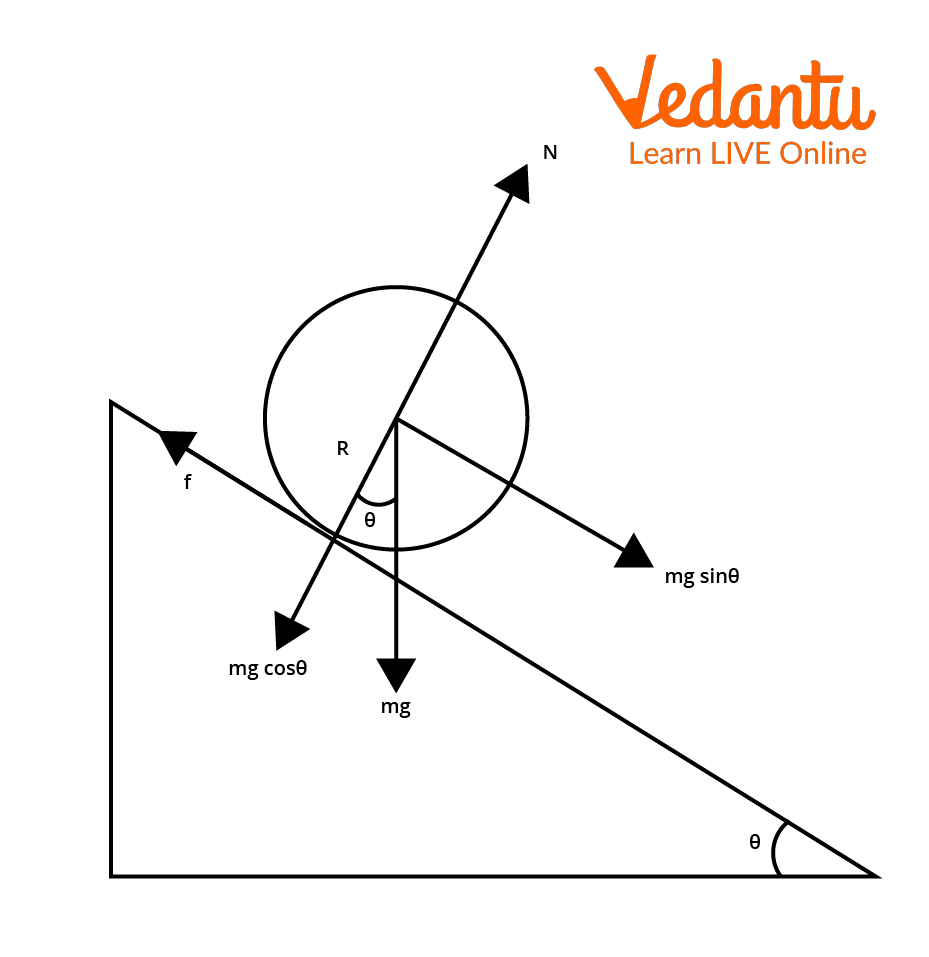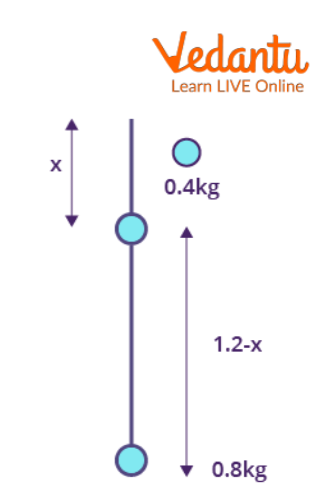Courses
Courses for Kids
Free study material
Free LIVE classes
More

# Pure Rolling on Inclined Plane and Its Formula for JEE## What is Pure Rolling Motion?

Last updated date: 25th Mar 2023
Total views: 72.3k
Views today: 1.49k

Pure rolling is defined as the absence of sliding during the rolling process. Pure rolling is a combination of translation and rotational motion, in which the point of contact comes to rest instantly because there is no sliding. The translational motion of a body is the motion of its centre of mass. During a body's rolling motion, the surfaces in contact are temporarily deformed. A finite area of both bodies comes into contact with each other as a result of this deformation. This phenomenon has the overall effect of opposing motion through the component of the contact force parallel to the surface, resulting in friction.

### Derivation of Kinetic Energy for Pure Rolling MotionDiagram Illustrating Rolling Motion

Let $v_{cm}$ be the velocity of a disc-shaped body's centre of mass. Since the centre of mass for a rolling disc is at the geometric centre C, the velocity of the body or velocity of C is $v_{cm}$, which is parallel to the rolling surface. The rotational motion of the body occurs about its axis of symmetry. Thus, the velocity at any point P0, P1 or P2 of the body consists of two parts: translational velocity due to rotational motion and other is the linear velocity, where $v_r = r\omega\cdot \omega$ is the angular velocity of the rolling disc. At any point on the disc concerning the geometric centre C, vr is perpendicular to the radius vector.

Consider the disc's point P0 , vis in the direction opposite to vcm , and at this point $v_r = R\omega$, where R is the radius of the disc. As a result,  $v_{cm} = R\omega$ gives the condition for rolling without slipping for the disc. The sum of the kinetic energies of translational motion and rotation gives the kinetic energy of such a rolling body.

$K=\dfrac{1}{2} m v_{c m}^{2}+\dfrac{1}{2} I \omega^{2}$

Here,

m denotes the body's mass.

The rotational motion is denoted by vcm.

I represents the moment of inertia.

$\omega$ is the rolling body's angular velocity.

This is the pure rolling formula of kinetic energy.

## Pure Rolling on Inclined Plane

Assume a round object with mass m and radius R rolls down an inclined plane without slipping. Two forces act on the object as it moves along the inclined plane. One is the gravitational force component ($mg\sin{\theta}$), and the other is static frictional force (f). The plane's normal force (N) cancels out the other component of gravitational force ($mg\cos{\theta}$). We will write the equation for motion from the object's free body diagram (FBP) as the motion occurs along the incline. Here, the body is rolling down an inclined plane as shown in figure below.Rolling Motion on Inclined Plane Diagram

### Derivation of Formulas for Pure Rolling Motion on Inclined Plane

Using Image 2 as the reference to derive the formulas related to the pure rolling motion on an inclined plane, we have

For translational motion, the supporting force is $mg\sin{\theta}$, and the opposing force is

$mg\sin{\theta}-f=ma$ .

Take the torque with respect to the object's centre for rotational motion. As a result, while $mg\sin{\theta}$ cannot cause torque as it passes through it, the frictional force f can set the torque of Rf.

$R_f=l\alpha$

Using the relationship, $a = r\alpha$ and the moment of inertia I=mK2 :

$R_f=m K^{2} \dfrac{a}{R} ;~f=m a \dfrac{K^{2}}{R^{2}}$

Substituting above equation we get:

\begin{align} &m g \sin \theta-m a\left(\dfrac{K^{2}}{R^{2}}\right)=m a \\ &m g \sin \theta=m a\left(\dfrac{K^{2}}{R^{2}}\right)+m a \\ &\operatorname{So}, a\left(1+\dfrac{K^{2}}{R^{2}}\right)=g \sin \theta \end{align}

After rewriting it for acceleration, we get:

$a=\frac{g \sin \theta}{\left(1+\frac{K^{2}}{R^{2}}\right)}$

Using the third equation of motion for the inclined plane v2 = u2 + 2as we can also find the expression for the rolling object's final velocity. If the body begins to roll from rest, u = 0. When h is the incline's vertical height, the length of the incline S is:

\begin{align} &\mathrm{S}=\dfrac{\mathrm{h}}{ \sin \theta} \\ &v^{2}=2 \dfrac{g \sin \theta}{\left(1+\dfrac{K^{2}}{R^{2}}\right)}\left(\dfrac{h}{\sin \theta}\right)=\dfrac{2 g h}{\left(1+\dfrac{K^{2}}{R^{2}}\right)} \\ &v=\sqrt{\dfrac{2 g h}{\left(1+\dfrac{K^{2}}{R^{2}}\right)}} \end{align}

The time required to roll down the incline could also be written as v = u + at from the first equation of motion. If, u = 0 for the object that begins rolling from rest. Then:

\begin{align} &t=\dfrac{v}{a} \\ &t=\sqrt{\dfrac{2 g h}{\left(1+\dfrac{K^{2}}{R^{2}}\right)}}\left(\dfrac{\left(1+\dfrac{K^{2}}{R^{2}}\right)}{g \sin \theta}\right) \\ &t=\sqrt{\dfrac{2 h\left(1+\dfrac{K^{2}}{R^{2}}\right)}{g \sin ^{2} \theta}} \end{align}

According to the equation, for a given incline, the object with the smallest radius of gyration K will arrive at the bottom of the incline first. Hence, the above mentioned is an inclined plane formula of time period.

We can also write the condition for pure rolling to avoid slipping $f \leq \mu_sN$

Here:

$\mu_s$ is the coefficient of friction.

\begin{align} &\dfrac{m g \sin \theta}{1+\dfrac{R^{2}}{K^{2}}} \leq \mu_{s} m g \cos \theta \\ &\mu_{s} \geq \dfrac{\tan \theta}{1+\dfrac{R^{2}}{K^{2}}} \end{align}

This is the condition of $\mu_s$ so that the body rolls without slipping.

## Applications of Pure Rolling Motion

The use of rolling-element bearings, such as ball bearings in rotating devices, is one of the most practical applications of rolling objects. The rolling elements are usually made of metal and are encased between two rings that can rotate independently of each other. The inner ring is usually attached to a stationary shaft in most mechanisms (or axle). As a result, while the inner ring remains stationary, the outer ring is free to move with minimal friction. This is the foundation upon which almost all motors (such as those found in ceiling fans, cars, and drills) operate. The amount of friction on the mechanism's parts is determined by the quality of the ball bearings and the amount of lubrication present.

## Solved Examples on Pure Rolling Motion

Example 1: Two-point masses of 0.4 kg and 0.8 kg are fixed at the ends of a rod which is of length 1.2 m and of negligible mass. The rod is set rotating about an axis perpendicular to its length with a uniform angular speed. The point on the rod through which the axis should pass in order that the work required for rotation of the rod is minimum is located at a distance of____.

Solution:

Given:Question Diagram

The moment of inertia of the system about the axis of rotation is

\begin{align}I &=I+I_{2} \\ & I=(0.4) x^{2}+(0.8)(1.2-x)^{2} \\ & I=(0.4) x^{2}+(0.8)\left(1.44+x^{2}-2.4 x\right) \\ & I=1.2 x^{2}+1.152-1.92 x \end{align}

The work done in rotating the rod is converted into its rotational kinetic energy.

\begin{align} W &=\dfrac{1}{2} I \omega^{2} \\ & W=\dfrac{1}{2}\left(1.2 x^{2}+1.152-1.92 x\right) \omega^{2} \end{align}

For the work done to be minimum,

\begin{align} &\dfrac{d W}{d x}=0 \\ &\text { i.e. } 2.4 x-1.92=0 \\ &x=\dfrac{1.92}{2.4} \\ &x=0.8 m \end{align}

Hence, The point on the rod through which the axis should pass in order that the work required for rotation of the rod is minimum is located at a distance of 0.8m.

Example 2: A circular disc of mass m and radius r is rolling on a smooth horizontal surface with a constant speed v. Calculate the kinetic energy of the disc.

Solution:

Given: A circular disc

Kinetic energy of the rolling disc is given by

$K=\dfrac{1}{2} I \omega^{2}+\dfrac{1}{2} m v^{2}$

For a disc, we have

$I=\dfrac{1}{2} m r^{2}$ as, $\omega=\dfrac{v}{r}$

So,

\begin{align} &K=\dfrac{1}{2}\left(\dfrac{1}{2} m r^{2}\right)\left(\dfrac{v}{r}\right)^{2}+\left(\dfrac{1}{2} m v^{2}\right) \\ &K=\left(\dfrac{1}{4} m v^{2}\right)+\left(\dfrac{1}{2} m v^{2}\right) \\ &K=\left(\dfrac{3}{4} m v^{2}\right) \end{align}

Hence, the kinetic energy of the disc is $\left(\dfrac{3}{4} m v^{2}\right)$.

## Conclusion

In short, pure rolling is the process of rolling where no sliding is visible. Pure rolling is a combination of translation and rotation motion in which the point of contact comes to rest instantly as there is no sliding. The sum of the kinetic energies of translational motion and rotation gives the kinetic energy of such a rolling body. As a result, this article aids us in better understanding the subject and goes into great detail about rolling motion particles.

## FAQs on Pure Rolling on Inclined Plane and Its Formula for JEE

1. A hollow sphere, a solid sphere and a ring are released from top of an inclined plane (frictionless) so that they slide down the plane. Which body would be in maximum acceleration?

When a body rolls down a plane, friction plays an important role. Also, friction depends on the mass of the body and basically opposes the action of sliding down a body. However, as given in the question, the plane is frictionless; so there will be no rolling motion and the masses will only slide down which makes it a sliding motion. Here, the acceleration $a=g\sin{\theta}$ . It is independent of the mass of the bodies. So, all the bodies will slide down with the same acceleration.

2. What are some of the factors that influence rolling resistance?

The following factors influence rolling resistance:

• Mass: The greater the mass of a body, the greater the downward force due to gravity. As a result, there is more compression, which raises rolling resistance.

• Cornering: When a rolling body, such as a bicycle, has to go around a corner, it slows down. This is due to increased rolling resistance caused by increased downward force on the cycle track while turning the corner.

• Tyre Design: If the tyre construction material used ensures less heating due to friction, there will be less rolling resistance.